# Scheduling for Drip Irrigation

### Summary

Probe for Windows v1.0.218+ has some additional features for users with drip irrigation.

(1) The required irrigation amount in the next week can be calculated from `DWU * 7 days * Factor`.
DWU can be ProbeDWU, or ET0 from a weather station. Factor is for RDI.
Configure on

(2) Irrigations and rainfalls can be posted to keydata rows. ProbeDWU will be calculated using these values.
Configure with Irrigation tab, and Gains Options/'Spray'

Other changes allow for calculation of effective gain if there are multiple gain events between readings, and irrigations amounts on the readings screen to be in mm, volume, time, or volume/emitter.

### Introduction

If water is being applied at frequent intervals soil moisture readings cannot be used to calculate a crop water use. The amount delivered could be exactly balanced by the crop water use, and the soil moisture will not change. Continuous moisture measurements will also have the same problem. In addition, because irrigations are so frequent, it may be appropriate to record weekly irrigation total amounts in keydata rows instead of posting to separate columns.

### Calculation of required amount

Use and choose 'drip' to calculate the amount required over the next few days from...

`Amount = Days * DWU`

Where `DWU` is the crop water use, and `Days` is the number of days that the water is to be delivered over (typically the number of days between readings)

e.g. Amount = 7 days * 5.0 mm/day = 35.0 mm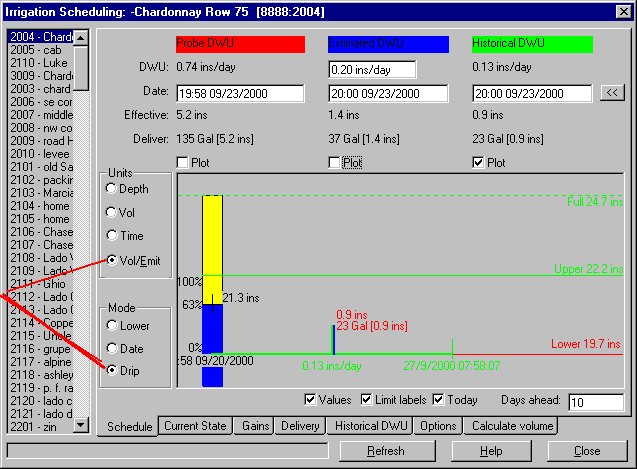In addition there are various optional corrections that can also be used.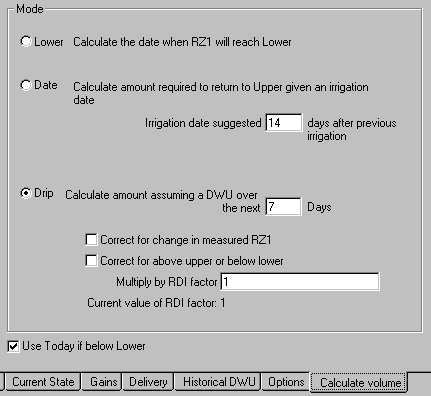#### Correction for change in RZ1

An optional correction can be applied to compensate for any rise or fall in measured soil moisture.

CorrectedAmount = Amount - (CurrentRZ1 - PreviousRZ1)

For example, if the soil moisture has risen from 245 to 250 over the last 7 days...

Amount = 35.0 - (250 - 245) = 30.0 mm

#### Correction for Outside Limits

An optional correction can be applied if the moisture content is above the upper or below the lower limits. (Upper=260, Lower=240 in the examples below).

If CurrentRZ1 is below LowerLimit then...

CorrectedAmount = Amount + (LowerLimit - CurrentRZ1)

e.g. Amount = 30.0 + (260 - 250) = 40.0 mm

If CurrentRZ1 above UpperLimit then...

CorrectedAmount = Amount - (CurrentRZ1 - UpperLimit)

Amount = 30.0 - (250 - 240) = 20.0 mm

The limit formulas are set on the CurrentState tab. They can be constant or they can be fomulas that vary during the seasons...

Limit Formula Result
`UPPER: FULL-10` 10 mm below full point
`LOWER: FULL-30` 30 mm below full point
`UPPER: FULL-GRAPES.HI` varying deficit in file GRAPES.LO
`LOWER: FULL-GRAPES.LO` varying deficit in file GRAPES.LO

By setting appropriate upper and lower limits the root zone water content should automatically track between the limits during the season.

#### RDI correction

Instead of using the upper and lower limits, the amount can simply be multiplied by an RDI factor

CorrectedAmount = Amount * RDIFactor

Amount = 40.0 * 0.4 = 16.0 mm

The amount to be delivered is 40% of the amount needed to supply the 'normal' crop water use.

The RDI factor can be entered as a constant (e.g. `0.4`) or a formula.

A formula allows you to control many sites from a single RDI factor.

For example, on all sites growing red grapes that have the same RDI factor enter...

RDI Factor: `RDIRED`

And on enter...

`RDIRED = 0.4`

The value used by each site would be 0.4, but this could be changed during the season.

#### Efficiency Factor

A site efficiency factor can be set on the Delivery tab to adjust for the wetted area. With a wetted area of say 20% of the total a `Factor=0.2` would be appropriate.

If this factor is required (called `FACTOR2`)it needs be entered in the text or graph report definition file.

For example, `GIPAMT` will be the amount in mm calculated from `HistoricalDWU*Days`, plus any corrections. In the irrigation requests (or graph) report the formula to include the efficiency factor will be...

`GPIAMT * FACTOR2`

#### Daily Water Use

Three different values of DWU are used to calculate three different water requirements...

 1. ProbeDWU water use as measured in the soil, calculated by Prwin 2. EstimatedDWU entered by user, but initial value from historical formula 3. HistoricalDWU from a formula, which could be long term values, or from a Penman-type calculation from the current weather e.g. CIMIS.

Examples of Historical DWU formulas...

 DWU formula Result `SHIRAZ.DWU*0.9` DWU from a season file of long term expected daily water use of grapes, multiplied by a crop factor. SHIRAZ.DWU can be edited with ProbeMenu/SeasonFiles `PETCIMIS*0.4` A value of DWU calculated fom the CIMIS weather station at Petaluma, multiplied by a crop factor (`0.4`) to allow for row spacing. `PETCIMIS=1.3` needs to be entered each week on ProbeMenu/Formulas.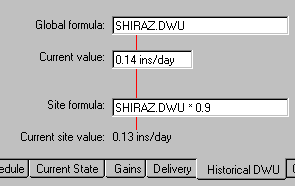### Posting Irrigations and Rainfalls to Keydata Rows

When multiple irrigations are applied between readings, some users have been entering the amounts in keydata rows instead of posting to separate columns in the readings screen.

Prwin (v1.0.218+) can now post irrigations and rainfall (gains) to keydata rows, and will also use these values to calculate Probe DWU. If the gain date is the same as a probe column the amounts will appear in the probe column, if the date is different the gain will have its own 'keydata' column.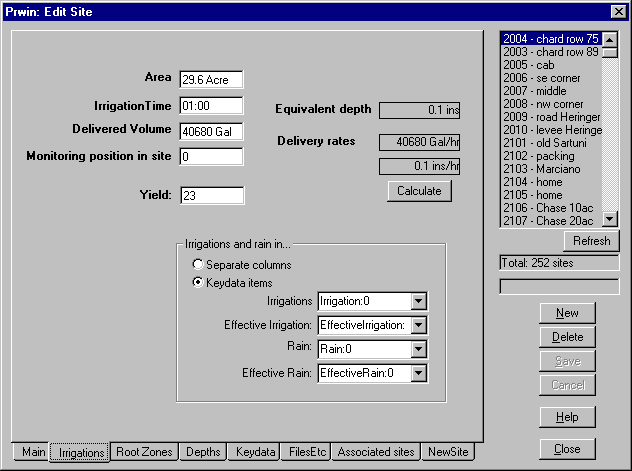Use to configure each site. On the keydata tab enter items for Irrigation, IrrigationEffective, Rain and RainEffective (effective amounts are optional). On the irrigations tab choose 'gains in keydata rows' and select the rows for the irrigation and rainfall amounts (effective rows are optional). Existing sites with irrigations already entered in keydata rows can be changed to the new method, but will be required after making the change.

The amounts will normally be displayed on the readings screen in mm or ins (as selected on ), but with you can choose to display the amounts in mm, volume, time, or volume per emitter.

#### Calculation of ProbeDWU

To calculate Probe DWU the total effective gain between probe readings is required. Prwin assumes that the amounts in a probe column were applied before the probe date, and amounts in any 'keydata' columns are also included.

ProbeDWU is calculated in the standard way...

`PDWU = (Current - Previous + EffectiveRain + EffectiveIrrigation) / Days`

If an effective row has not been specified the rain and irrigation amounts will be the delivered amounts and not the effective amounts.

To calculate ProbeDWU from gains in keydata rows you must choose the 'ConstantDWU' option on ProbeMenu/Update Gain Options tab.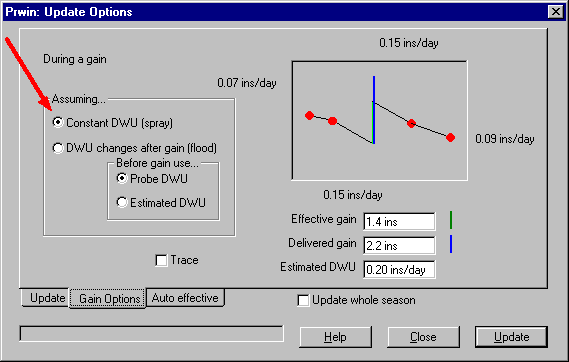#### Automatic calculation of effective amounts

If keydata rows for effective amounts have also been specified the effective gain will be automatically calculated in the same way as irrigations posted to separate columns.

To re-calculate the effective amount, on the readings screen a 'right-click' on a probe column with irrigations or rainfalls in keydata rows will show a popup menu to re-calculate the effective irrigation and rainfall amounts. If the right click was on...

Row Result
Irrigation Re-calculate the effective irrigation, but not the effective rainfall.
Rain Re-calculate the effective rainfall, but not the effective irrigation.
neither irrigation or rainfall Re-calculate both, assuming the irrigation and rainfall have the same efficiency.

If gains are posted to separate columns, and there is more than one gain (irrigation or rainfall) column between probe readings, a right-click on a column will...

Column Result
Irrigation or rainfall Re-calculate the effective amount for just that column.
Estimate Re-calculate all columns spreading the efficiency equally to all the gains in this period.

#### Posting gains using ProbeMenu/Post Irrigations and Rainfalls

Gains will only be posted to the keydata row of a Probe column if the probe readings are posted first. If there is no probe column with the same date as the gain the gain will posted to a separate keydata column.

#### Posting Gains using ProbeMenu/PostReadings

If gains are posted at the same time as posting readings the option 'post to same column' should be chosen.

### Example of predictions for drip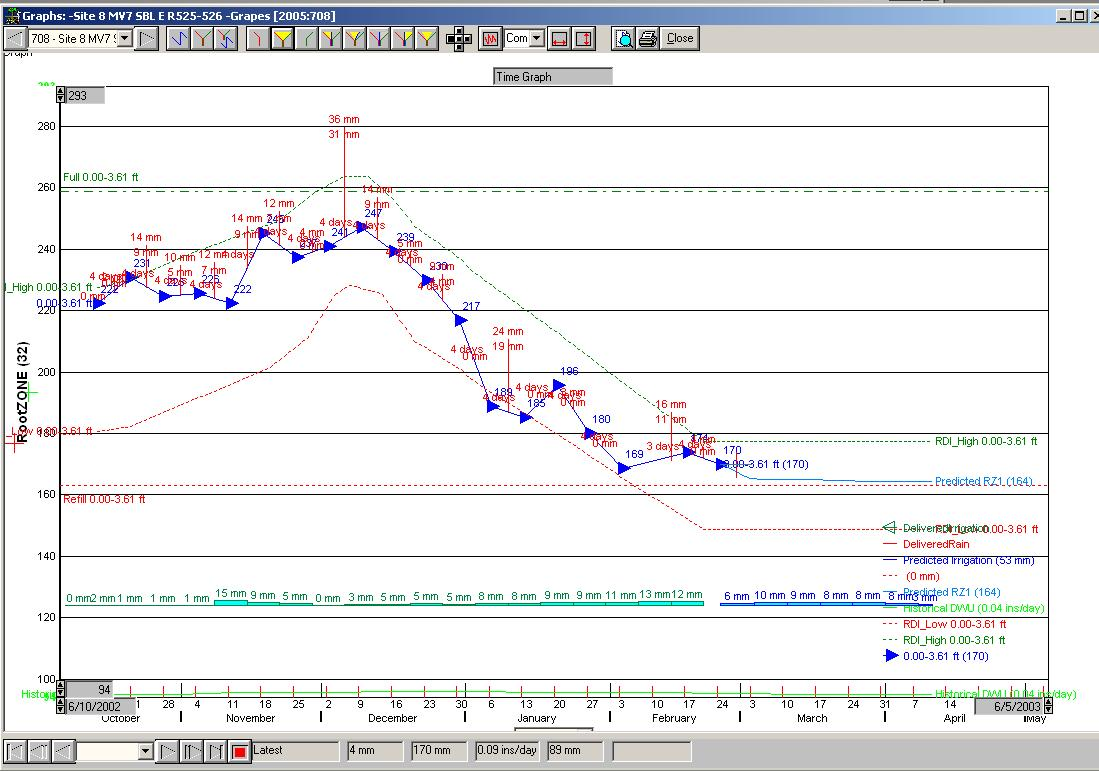The dark blue line and symbols are measured soil moisture. The extension in pale blue is the calculated soil moisture, with a target mid-way between the upper and lower limits.

The calculated water requirements take into account a varying target soil moisture; a varying crop ET rate; and assumed rainfalls from a 'previous season'.

The applied drip amounts each week are at the bottom of the graph - actual amounts applied in pale green, predicted requirements in darker green.

The target upper and lower limits are varying during the season to implement an RDI strategy.